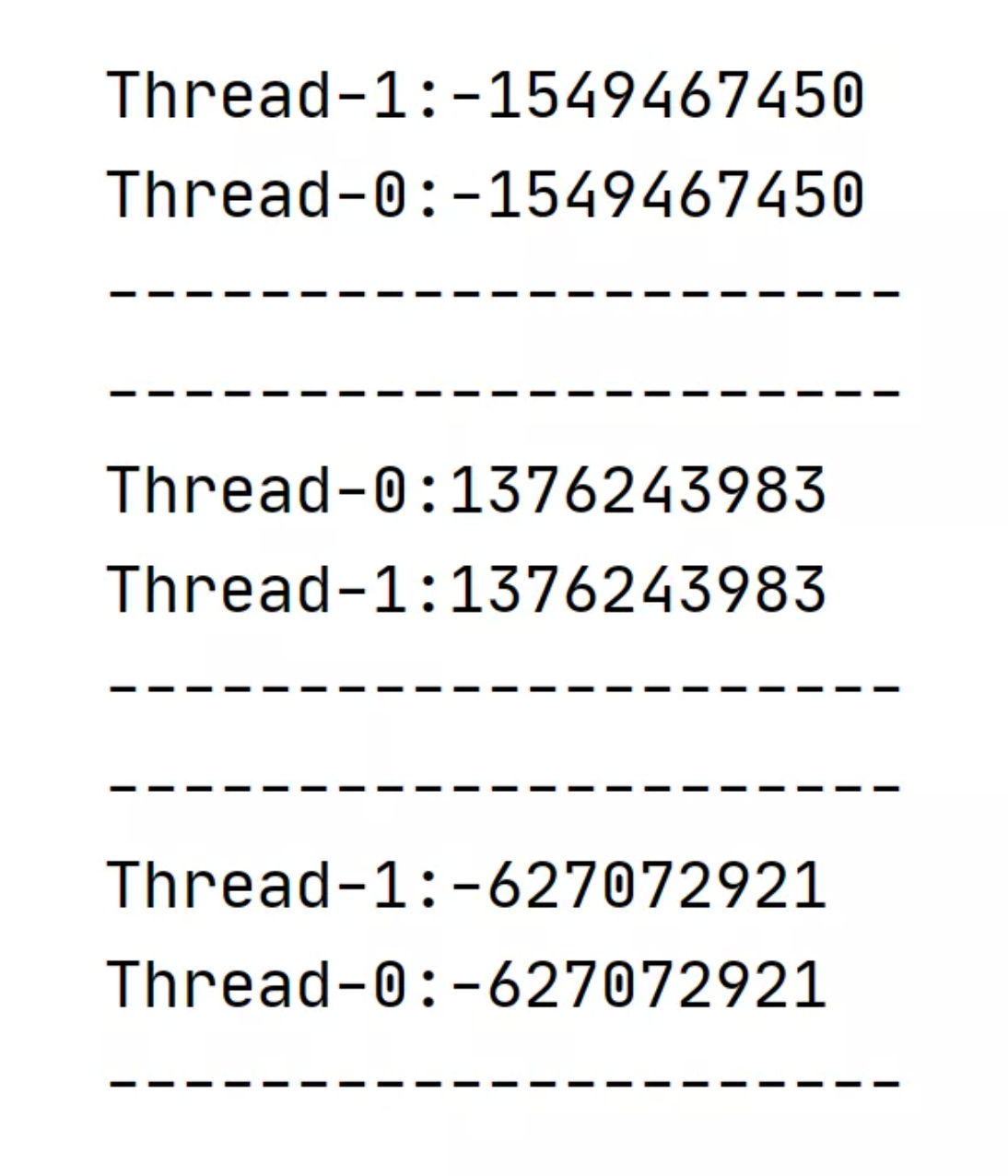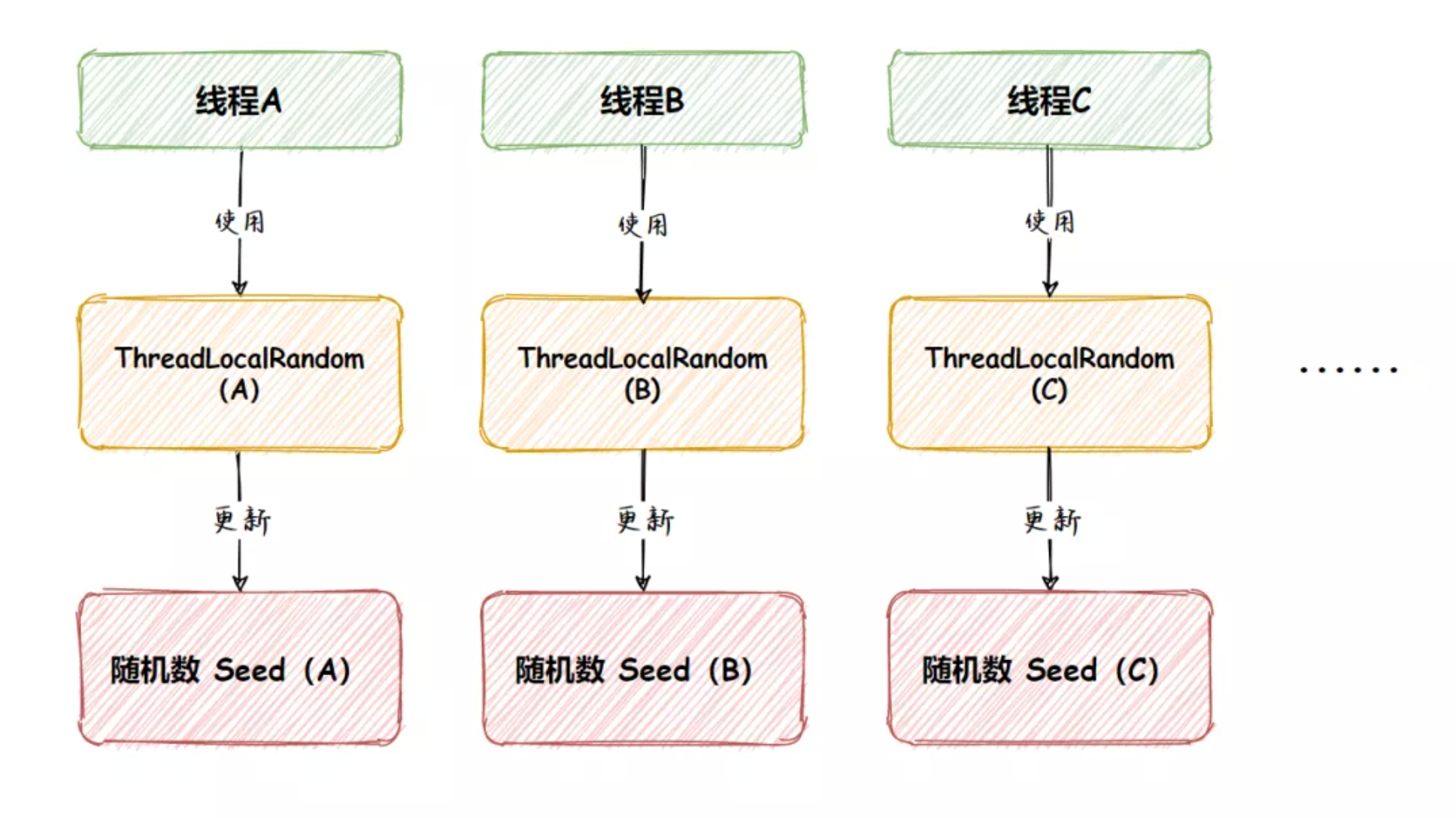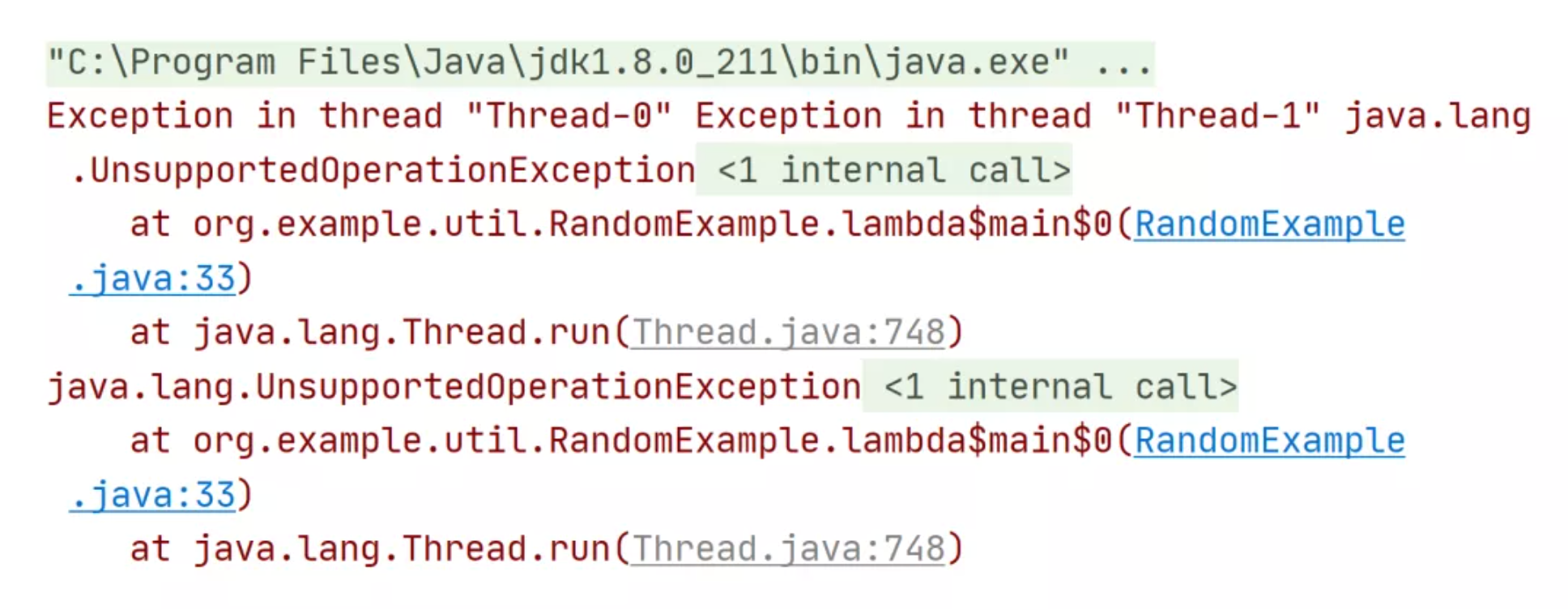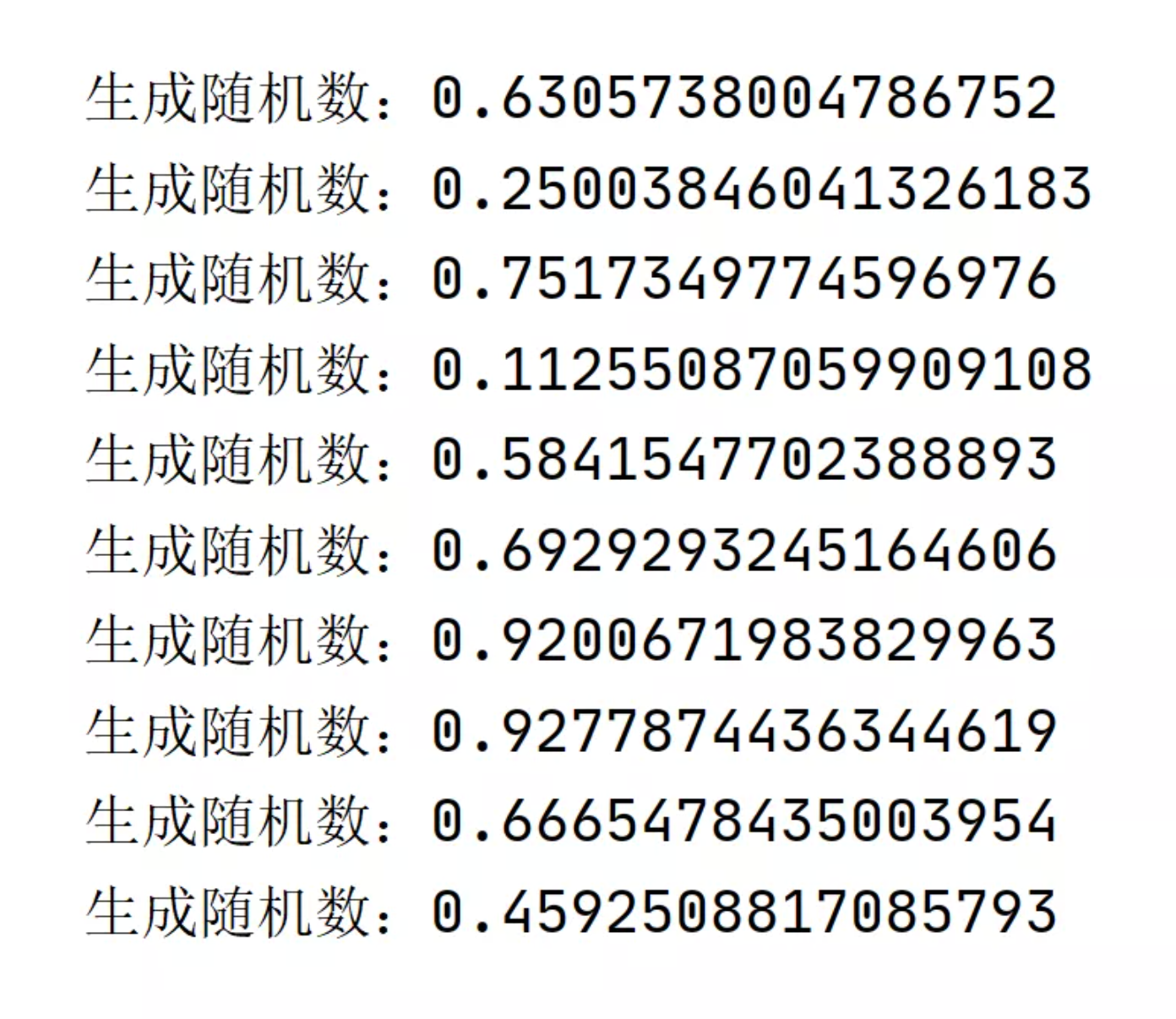# Java 四种生成随机数方式

2021/07/16 14:13

1、Random

Random 类诞生于 JDK 1.0，它产生的随机数是伪随机数，也就是有规则的随机数。Random 使用的随机算法为 linear congruential pseudorandom number generator (LGC) 线性同余法伪随机数。在随机数生成时，随机算法的起源数字称为种子数（seed），在种子数的基础上进行一定的变换，从而产生需要的随机数字。

Random 对象在种子数相同的情况下，相同次数生成的随机数是相同的。比如两个种子数相同的 Random 对象，第一次生成的随机数字完全相同，第二次生成的随机数字也完全相同。默认情况下 new Random() 使用的是当前纳秒时间作为种子数的。

1.1、基础使用

// 生成 Random 对象
Random random = new Random();
for (int i = 0; i < 10; i++) {
// 生成 0-9 随机整数
int number = random.nextInt(10);
System.out.println("生成随机数：" + number);
}1.2、优缺点分析

Random 使用 LGC 算法生成伪随机数的优点是执行效率比较高，生成的速度比较快。

// 创建两个线程
for (int i = 0; i < 2; i++) {
// 创建 Random 对象，设置相同的种子
Random random = new Random(1024);
// 生成 3 次随机数
for (int j = 0; j < 3; j++) {
// 生成随机数
int number = random.nextInt();
// 打印生成的随机数
number);
// 休眠 200 ms
try {
} catch (InterruptedException e) {
e.printStackTrace();
}
System.out.println("---------------------");
}
}).start();
}1.3、线程安全问题

PS：线程安全指的是在多线程的场景下，程序的执行结果和预期的结果一致，就叫线程安全的，否则则为非线程安全的（也叫线程安全问题）。比如有两个线程，第一个线程执行 10 万次 ++ 操作，第二个线程执行 10 万次 -- 操作，那么最终的结果应该是没加也没减，如果程序最终的结果和预期不符，则为非线程安全的。

public Random() {
this(seedUniquifier() ^ System.nanoTime());
}

public int nextInt() {
return next(32);
}

protected int next(int bits) {
long oldseed, nextseed;
AtomicLong seed = this.seed;
do {
oldseed = seed.get();
} while (!seed.compareAndSet(oldseed, nextseed)); // CAS（Compare and Swap）生成随机数
return (int)(nextseed >>> (48 - bits));
}

PS：本文所有源码来自于 JDK 1.8.0_211。

PS：Java 并发机制实现原子操作有两种：一种是锁，一种是 CAS。

CAS 是 Compare And Swap（比较并替换）的缩写，java.util.concurrent.atomic 中的很多类，如（AtomicInteger AtomicBoolean AtomicLong等）都使用了 CAS 机制来实现。2.1、基础使用

// 得到 ThreadLocalRandom 对象
for (int i = 0; i < 10; i++) {
// 生成 0-9 随机整数
int number = random.nextInt(10);
// 打印结果
System.out.println("生成随机数：" + number);
}2.2、实现原理

public int nextInt(int bound) {
// 参数效验
if (bound <= 0)
// 根据当前线程中种子计算新种子
int r = mix32(nextSeed());
int m = bound - 1;
// 根据新种子和 bound 计算随机数
if ((bound & m) == 0) // power of two
r &= m;
else { // reject over-represented candidates
for (int u = r >>> 1;
u + m - (r = u % bound) < 0;
u = mix32(nextSeed()) >>> 1)
;
}
return r;
}

final long nextSeed() {
// 获取当前线程中 threadLocalRandomSeed 变量，然后在种子的基础上累加 GAMMA 值作为新种子
// 再使用 UNSAFE.putLong 将新种子存放到当前线程的 threadLocalRandomSeed 变量中
r = UNSAFE.getLong(t, SEED) + GAMMA);
return r;
}

2.3、优缺点分析

public void setSeed(long seed) {
// only allow call from super() constructor
if (initialized)
thrownew UnsupportedOperationException();
}private static long initialSeed() {
// 尝试获取 JVM 的启动参数
String sec = VM.getSavedProperty("java.util.secureRandomSeed");
// 如果启动参数设置的值为 true，则参数一个随机 8 位的种子
if (Boolean.parseBoolean(sec)) {
byte[] seedBytes = java.security.SecureRandom.getSeed(8);
long s = (long)(seedBytes) & 0xffL;
for (int i = 1; i < 8; ++i)
s = (s << 8) | ((long)(seedBytes[i]) & 0xffL);
return s;
}
// 如果没有设置启动参数，则使用当前时间有关的随机种子算法
return (mix64(System.currentTimeMillis()) ^
mix64(System.nanoTime()));
}

3、SecureRandom

SecureRandom 继承自 Random，该类提供加密强随机数生成器。SecureRandom 不同于 Random，它收集了一些随机事件，比如鼠标点击，键盘点击等，SecureRandom 使用这些随机事件作为种子。这意味着，种子是不可预测的，而不像 Random 默认使用系统当前时间的毫秒数作为种子，从而避免了生成相同随机数的可能性。

3.1、基础使用

// 创建 SecureRandom 对象，并设置加密算法
SecureRandom random = SecureRandom.getInstance("SHA1PRNG");
for (int i = 0; i < 10; i++) {
// 生成 0-9 随机整数
int number = random.nextInt(10);
// 打印结果
System.out.println("生成随机数：" + number);
}SecureRandom 默认支持两种加密算法：

1. SHA1PRNG 算法，提供者 sun.security.provider.SecureRandom；
2. NativePRNG 算法，提供者 sun.security.provider.NativePRNG。

SecureRandom secureRandom = new SecureRandom();

4、Math

Math 类诞生于 JDK 1.0，它里面包含了用于执行基本数xueyun算的属性和方法，如初等指数、对数、平方根和三角函数，当然它里面也包含了生成随机数的静态方法 Math.random() ，此方法会产生一个 0 到 1 的 double 值，如下代码所示。

4.1、 基础使用

for (int i = 0; i < 10; i++) {
// 产生随机数
double number = Math.random();
System.out.println("生成随机数：" + number);
}4.2、 扩展

for (int i = 0; i < 10; i++) {
// 生成一个从 0-99 的整数
int number = (int) (Math.random() * 100);
System.out.println("生成随机数：" + number);
}4.3、实现原理

public static double random() {
return RandomNumberGeneratorHolder.randomNumberGenerator.nextDouble();
}

privatestaticfinalclass RandomNumberGeneratorHolder {
staticfinal Random randomNumberGenerator = new Random();
}

5、总结0 评论
0 收藏
0